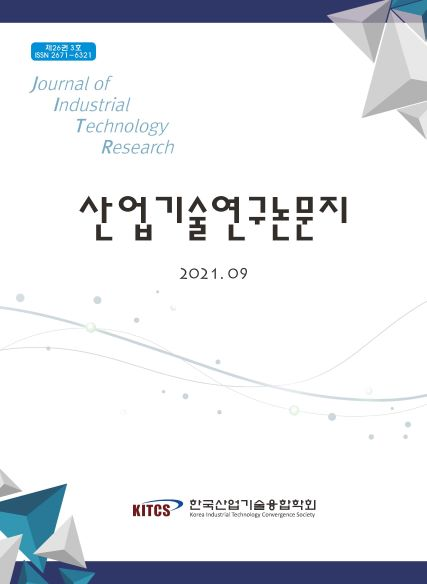상세검색
최근 검색어 전체 삭제
다국어입력
즐겨찾기0KCI등재 학술저널

# 기업의 최적 자본구조 결정요인에 관한 분석패널데이터를 중심으로

• 등재여부 : KCI등재후보
• 2021.09
• 77 - 87 (11 pages)
• 119

This study examined the existing theoretical and empirical studies on the determinants of capital structure. Based on the determinants identified, the periods before and after the financial crisis were compared and analyzed. The data were analyzed for the time series period 1991-2000, and 350 companies that were continuously listed during the period were analyzed. First, for the period 1991-2000, in the fixed effects model, the significant variables were the fixed ratio (t=39.782). In the random effects model, the fixed ratio (t=41.306) and total assets (-4.919) were significant variables. Second, in the period before the financial crisis (1991-1997), the fixed ratio in both the fixed (t=30.243) and random (t=32.016) effects models and total assets (-4.179) were significant variables. However, in the fixed and random effects models, the profitability (t=-1.958, t=-1.758) is meaningless at the 5% significance level but may be meaningful at the 10% significance level in the one-sided test. Third, in the period after the financial crisis (1998-2000), the fixed ratio in the fixed (t=14.860) and random (t=20.443) effects models and total assets (t=-3.286) were significant variables.

Ⅰ. 서 론

Ⅱ. 이론적 배경

Ⅲ. 변수 및 회귀 모형 설정

Ⅳ. 실증분석 결과

Ⅴ. 결론 및 향후 과제

참고문헌